# Crown

Siblings collect a 2-crowns and 5-crowns. Together have 80 coins with a total of value 310 crowns. How many saved two-crowns and how many five-crowns?

Correct result:

n(2) =  30
n(5) =  50

#### Solution:

a+b = 80; 2a+5b = 310

a+b = 80
2•a+5•b = 310

a+b = 80
2a+5b = 310

a = 30
b = 50

Calculated by our linear equations calculator.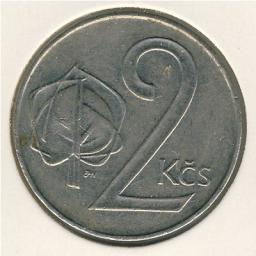Our examples were largely sent or created by pupils and students themselves. Therefore, we would be pleased if you could send us any errors you found, spelling mistakes, or rephasing the example. Thank you!

Please write to us with your comment on the math problem or ask something. Thank you for helping each other - students, teachers, parents, and problem authors.Tips to related online calculators
Do you have a linear equation or system of equations and looking for its solution? Or do you have quadratic equation?

## Next similar math problems:

• Two math problems1) The sum of twice a number and -6 is nine more than the opposite of that number. Find the number. 2) A collection of 27 coins, all nickels, and dimes, is worth \$2.10. How many of each coin are there? The dime, in United States usage, is a ten-cent coin.
• Home cleaning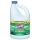Mr. Smith is cleaning up a big mess at home. In the closet, he finds a solution that is 5% bleach, and another stronger solution that is 20% bleach. For this particular job, he needs 600mL of 15% bleach. How much of each type (to the nearest mL) should he
• Mixing waterThe 30-liter container should we fill with water at 60 degrees Celsius. How many liters of water 80 degrees C hot and how many liters of water 20 degrees Celsius warm we have to mix?
• Alcohol mixtureFrom 55% and 80% alcohol we have to produce 0.2 kg of 60% alcohol. How many of them do we use in solution?
• BonbonsCreate a mixture of 50 kg of candy on price 700Kč. Candies has prices: 820Kč, 660Kč and 580Kč. Use cross rule.
• ConcentrationHow many grams of 65% and 50% acid is to be mixed to give 240 g acid with a concentration of 60%?
• CoinsThe money - coins are minted from the hardest bronze, which contains copper and tin in a ratio of 41: 9. How much copper and tin are in 2kg of bronze money?
• Sales of productsFor 80 pieces of two quality products a total sales is 175 Eur. If the first quality product was sold for n EUR per piece (n natural number) and the second quality product after 2 EUR per piece. How many pieces of the first quality were sold?
• Candies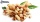In the confectionery, the price for 1 kg of pistachio candies cost CZK 360, and the price for 1 kg of hazelnut candies was CZK 280. Mixing these two types of sweets created a box of chocolates. How many grams of pistachios and how many grams of hazelnut c
• The pool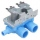The pool contains 220 m3 of water. The pool can be emptied either: a) 10 hours of pipe B and 8 hours of pipe A, or b) 10 hours of pipe A and 7 hours of pipe B. How many cubic meters of water will flow in 1 hour from pipe A and how many from pipe B?
• Beds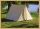At the summer camp, there are 41 chalets. Some rooms are 3-beds, some 4-beds. How many campers from 140 are living in 3-bed?
• Tea mixture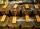Of the two sort of tea at a price of 180 CZK/kg and 240 CZK/kg we make a mixture 12 kg that should be prepared at a price of 200 CZK / kg. How many kilos of each sort of tea will we need to be mixed?
• Tulips and daffodils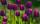Two hundred twenty tulips and daffodils are planted in the flowerbed. One-third of all tulips and one-sixth of all daffodils equals the number of all tulips. How many tulips and how many daffodils?
• SweetsWe want to prepare 5 kg of sweets for 150 CZK. We will mix cheaper candy: 1 kg for 120 CZK and more expensive candy: 1 kg per 240 CZK. How much of this two types of candy is necessary to prepare this mixture?
• CuZn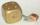Brass is an alloy of copper and zinc. The 10 centimeter brass cube has a weight of 8.6 kg. Copper density is 8930 kg/m3, the zinc density is 7130 kg/m3. Calculate how many kg of copper and zinc a cube contains.
• SolutionWhat amount of 80% solution and what amount of water is needed to mix in order to obtain two liters of 60% solution of this mixture?
• RingThe ring made from gold and copper alloy has a weight of 14.5 g and a volume of 1.03 cm3. How much gold and how much copper it contains? The metal densities are Au 19.3 g/cm³ and Cu 8.94 kg·dm-3